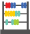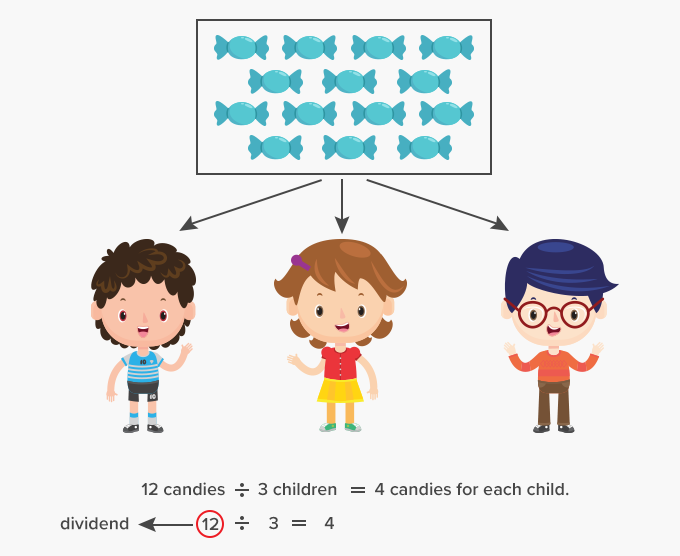# Dividend - Definition with Examples

The Complete K-5 Math Learning Program Built for Your Child

• 40 Million Kids

Loved by kids and parent worldwide

• 50,000 Schools

Trusted by teachers across schools

• Comprehensive Curriculum

Aligned to Common Core

##Let's learn!

What is a dividend?
In division, the amount or number to be divided is called the dividend.
Dividend is the whole that is to be divided into parts.

Here, for example, 12 candies are to be divided among 3 children. 12 is the dividend.In long division, the dividend is written inside or within the tableau drawn.Formula for finding the dividend is –

 Dividend = Divisor x Quotient + Remainder

 Fun Facts Dividends are also one of the ways in which companies “share the wealth” generated in the business.

##Let's sing!

Dividend is the total money you have to spend,
Or the total number of candies you have to send.
It is the whole you have to divide in the end!

##Let's do it!

Before handing out math division worksheets to your children, discuss the parts of a division problem – dividend, divisor, quotient and remainder. Ask children to identify the dividend in division equations and word problems.

##Related math vocabulary

Won Numerous Awards & Honors